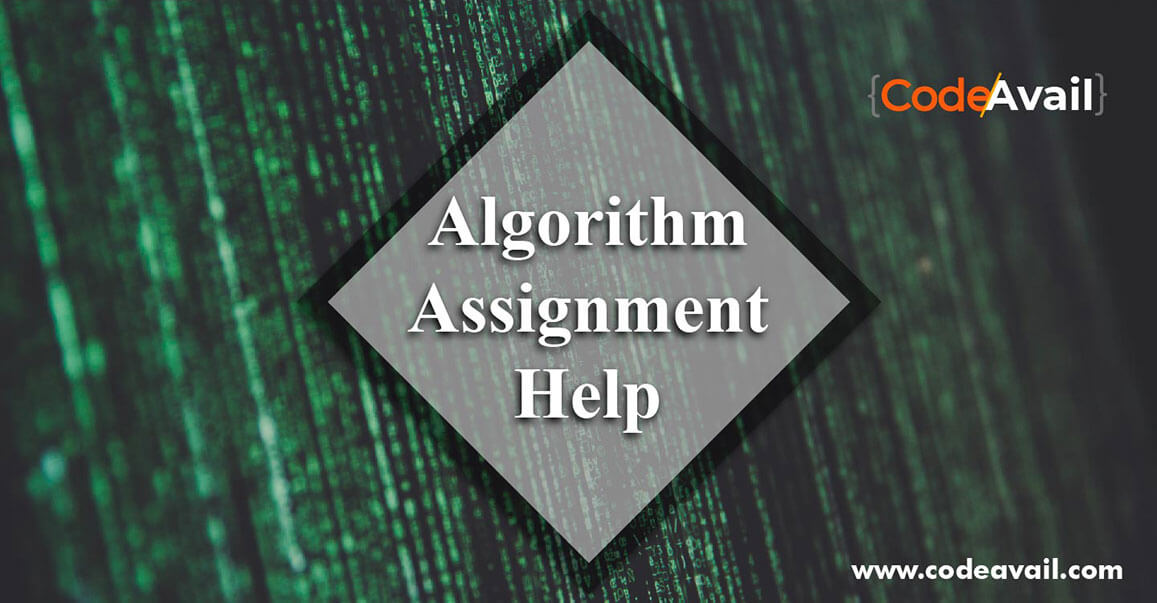# Algorithm Assignment Help By CS Experts

We provide you with algorithm assignment help, algorithm homework help, and programming algorithm help in every topic of your programming language.

With a deep understanding of algorithms and its core concepts, our programming algorithm assignment experts will help you define basic and algorithms based on your programming assignment theory. We can help you create efficient and accurate algorithms to achieve the goal of your Application or Operation.## What is the Programming Algorithm?

In simple words, an algorithm is a group of commands designed to perform a particular task. There are many ways to accomplish any task, especially in the programming language. So programmers design the most efficient algorithms that increase the speed to run programs.

## Why students need Programming Algorithm Homework Help?

It helps in deciding according to parameters and choosing the values on the other. Many students find it difficult to solve algorithm assignments and arrive at proper algorithm solutions. Many students often neglect the algorithms given by their high school, college or university teachers to do the homework. Our experts at Codeavail help you in all computer science programming algorithm assignment.

When writing a good algorithm, it should be written in such a way that it can be applied in all programming languages. Algorithms may be common to many programming languages to obtain the same output.

Every step in the algorithm should be clear and very important. The algorithms in programming should have a clear start and a stopping point. Some students usually make the mistake of the algorithm with coding but our experts provide you the algorithm solution to get an expected result and to score higher in your algorithm assignment or homework.

## Points to be remembered for a good programming algorithm

There are various ways to classify algorithms. Some popular ways are:

• Every step in algorithms is important. So, each step should be clear and well organized.
• Use the syntax where necessary.
• Between different methods of giving a directive, the algorithms used should be effective.
• Mention a sub-number list if there are any loops present in the algorithm.
• In programming, include variables and also their use.
• Input and output should be accurately defined.
• The algorithm should be designed in such a way that it can be applied in more than one programming language.
• Algorithm should have a finite number of execution of the commands or instructions.

## Programming algorithm to multiply two numbers and display the result

step1-START

step2-declare three integers a,b&c

step3-define values of a&b

step4–multiply values of a&b

step5-store output of step 4to c

step6-printc

step7-STOP

## Basics of the Programming Algorithm

Students are not clear with the basics. Thus, they find algorithms difficult to do homework and assignments; Without proper algorithms, students are helped to ignore the subject; We believe in the step-by-step guidance of students in algorithm assignments to ensure that they understand the concept properly and do homework of algorithms. Therefore, they do not face the above-mentioned problems and develop a healthy approach to algorithmic assignments in the future.

## Programming Algorithm Problem Solving Paradigms

Some of the popular tools/approaches are:-

Complete Search/Brute Force:

A method is that in which we look at all the chances and selects the best solution.

Pros: Simple should always find solutions because you are looking at every possibility.

Cons: As the number of possibilities increases the number of objects increases as fast as possible.

Divide and Conquer:

In this method that we divide the problem into smaller parts and then solve those parts which are very easy to solve. Think binary search.

Greedy Approach:

The method that keeps the best option at the current time, without any concern for the future.

Pros: Can get quick, simple, or best solutions.

Cons: Most of the time, we will not receive the best solution.

Dynamic Programming:

A method that is created to the solution using the sub-solutions that were previously found. Certainly one of the more advanced techniques, but extremely powerful and applicable.

Pros: Polynomial finds the optimal solution of many problems in time (while brute force will take faster)

Cons: Understanding and implementing is difficult, it takes time to understand different states and recurrence.

## We provide help for Topics like:

-Dynamic Programming, Elementary geometric algorithms,fingerprinting, network flow, amortization, randomization.

-Analysis, implementation, Searching Algorithms, Linear programming, fixed-parameter algorithms,

-Streaming algorithms, and data structures, approximation algorithms, Programming in C++ , Elementary Data Structure, online algorithms.

-Randomized algorithms, -probabilistic analysis, heuristics, online algorithms, parallel algorithms, number-theoretic algorithms, network algorithms, VLSI CAD algorithms

## Our Experts provide help in these Important topics in Programming Algorithm and furthermore which are included:

• General Combinatorial Algorithm Solution
• Graph Algorithm Solution
• Number Theoretic Algorithm Solution
• Optimization Algorithm Solution
• Database Algorithm Solution
• Operating Systems Algorithm Solution
• Distributed Systems Algorithm Solution